# Abstract

In this paper a trigonometric shear deformation theory is presented for the free vibration of thick orthotropic square and rectangular plates. In this displacement based theory the in-plane displacement field uses sinusoidal function in terms of thickness coordinate to include the shear deformation effect. The cosine function in terms of thickness coordinate is used in transverse displacement to include the effect of transverse normal strain. The most important feature of the theory is that the transverse shear stress can be obtained directly from the constitutive relations satisfying the shear stress free surface conditions on the top and bottom surfaces of the plate. Hence the theory obviates the need of shear correction factor. Governing equations and boundary conditions of the theory are obtained using the principle of virtual work. Results obtained for frequency of bending mode, shear mode and thickness stretch mode of free vibration of simply supported orthotropic square and rectangular plates are compared with those of other refined theories and exact solution from theory of elasticity wherever applicable.

shear deformation; thick orthotropic plate; transverse normal strain; free vibration; frequencies

Free vibration of thick orthotropic plates using trigonometric shear deformation theory

Y. M. Ghugal* * Author email: ghugal@rediffmail.com ; A. S. Sayyad

Department of Applied Mechanics, Government Engineering College, Aurangabad-431005, Maharashtra State, India

ABSTRACT

In this paper a trigonometric shear deformation theory is presented for the free vibration of thick orthotropic square and rectangular plates. In this displacement based theory the in-plane displacement field uses sinusoidal function in terms of thickness coordinate to include the shear deformation effect. The cosine function in terms of thickness coordinate is used in transverse displacement to include the effect of transverse normal strain. The most important feature of the theory is that the transverse shear stress can be obtained directly from the constitutive relations satisfying the shear stress free surface conditions on the top and bottom surfaces of the plate. Hence the theory obviates the need of shear correction factor. Governing equations and boundary conditions of the theory are obtained using the principle of virtual work. Results obtained for frequency of bending mode, shear mode and thickness stretch mode of free vibration of simply supported orthotropic square and rectangular plates are compared with those of other refined theories and exact solution from theory of elasticity wherever applicable.

Keywords: shear deformation, thick orthotropic plate, transverse normal strain, free vibration, frequencies.

1 INTRODUCTION

The use of composite materials has increased steadily during last two decades, particularly in aerospace, underwater and automotive structures. This is largely because many composite materials exhibit high strength-to-weight and stiffness-to-weight ratios, which make them ideally suited for use in weight-sensitive structures.

The study of free vibration dates back to the 1800s. Kirchhoff [9, 10] developed classical plate theory (CPT) which is based on hypothesis that straight lines normal to the undeformed midplane remain straight and normal to the deformed midplane. Since the transverse shear deformation is neglected in Kirchhoff theory, it can not be adequate for the analysis of shear flexible thick plates wherein shear deformation effects are more significant. Thus, its suitability is limited to only thin plates; as a consequence it under predicts deflections and over predicts natural frequencies.

First order shear deformation theories (FSDTs) can be considered as improvements over classical plate theory. It is based on the assumption that straight lines normal to undeformed midplane remain straight but not necessarily normal to the deformed midplane. Mindlin et al.  investigated the free flexural vibration of rectangular plate. Reissner  was the first to develop a theory which incorporates the effect of shear. Reissner's formulation comes out as a special case of Librescu's  approach.

Chladni  studied the free vibration of a square plate with completely free edges. Rayleigh  presented his well-known general method of solution for the natural frequencies of vibration. Ritz  improved the Rayleigh procedure by assuming a set of admissible trial functions. Levinson  has developed a displacement based theory which does not require shear correction factor. The governing equations for the motion of a plate obtained by Levinson's approach are same as those by Mindlin's theory, provided that the shear coefficient value associated with the Mindlin's theory is taken as 5/6.

Many higher order theories are available in the literature for the static flexure and free vibration analysis of thick plates e.g., theories by Nelson and Lorch  with nine unknowns, Krishna Murty  with 5, 7, 9. . . unknowns, Lo et al. [16, 17] with 11 unknowns, Kant  with six unknowns, Bhimaraddi and Stevens  with five unknowns, Reddy  with eight unknowns, Hanna and Leissa  with four unknowns. Srinivas et al.  used an exact three dimensional plate theory to study the vibration of simply supported homogenous and laminated thick rectangular plates.

Levy  has developed a refined theory for thick plate for the first time using sinusoidal functions in the displacement field. Stein  has used theory using trigonometric functions for analysis of laminated beams and plates. A critical review of the plate theories has been given by Vasil'ev  and Noor and Burton . Whereas Liew et al.  surveyed plate theories particularly applied to thick plate vibration problems. A recent review paper is presented by Ghugal and Shimpi . Shimpi and Patel  have developed a two variable refined plate theory for the free vibration of orthotropic plate; however theory overestimates the results of bending frequencies compared to those of exact theory. Kim and Reddy  have developed novel mixed finite element models for nonlinear analysis of plates based on the classical and first order shear deformation theories.

In this paper a displacement based trigonometric shear deformation theory is presented for the free vibration of orthotropic square and rectangular plates which includes effect of transverse shear and transverse normal strain.

2 PLATE UNDER CONSIDERATION

Consider a plate (of length a, width b, and thickness h) of homogenous material. The plate occupies (in O  x  y  z right-handed Cartesian coordinate system) a region2.1 Assumptions made in theoretical formulation

1. The displacement components u and v are the inplane displacements in x and y  directions respectively and w is the transverse displacement in z-direction. These displacements are small in comparison with the plate thickness.

2. The in-plane displacement u in x -direction and v in y -direction each consist of two parts:

a. a displacement component analogous to displacement in classical plate theory of bending;

b. displacement component due to shear deformation which is assumed to be sinusoidal in nature with respect to thickness coordinate.

3. The transverse displacement w in z -direction is assumed to be a function of x, y and z coordinates.

4. The body forces are ignored in the analysis.

5. The plate is subjected to transverse load only.

2.2 The displacement field

Based upon the before mentioned assumptions, the displacement field of the present plate theory is given as below:where u and v are the inplane displacements in x and y directions respectively and w is transverse displacement in z -direction. The sinusoidal function is assigned according to the shear stress distribution through the thickness of the plate. The φ, ψ and ξ represent rotations of the plate at neutral surface, which are unknown functions to be determined.

2.3 Strain displacement relationship

Normal and shear strains are obtained within the framework of linear theory of elasticity using displacement field given by Eq. (2).

Normal strains:Shear strains: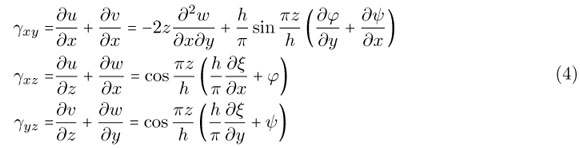2.4 Stress-strain relationship

The following stress-strain relationships are used to obtain normal and transverse shear stresses.where [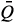ij] are the reduced stiffness coefficients as given by Jones  are as follows.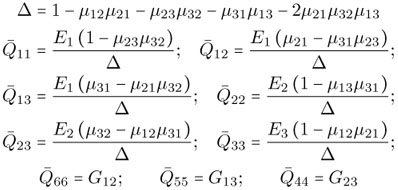2.5 Derivation of governing equations and boundary conditions

Using Eq. (3) through (5) and dynamic version of principle of virtual work, variationally consistent differential equations and boundary conditions for the plate under consideration are obtained. The dynamic version of principle of virtual work when applied to the plate leads to:Employing Green's theorem in Eq. (6) successively, we obtain the coupled Euler-Lagrange governing equations of the plate and the associated boundary conditions of the plate in terms of stress resultants. The governing differential equations are as follows:The boundary conditions at x = 0 and x = a obtained are of the following form:and along y = 0 and y = b edges, the boundary conditions are as follows:At corners (x = 0, y = 0), (x = a, y = 0), (x = 0,y = b), (x = a, y = b) boundary condition is: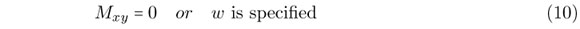Here the stress resultants appear in the governing equations and boundary conditions are defined as follows: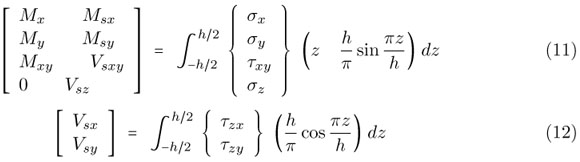where Mx, My, Mxy are moment resultants analogous to classical plate theory, Msx, Msy are refined moments due to transverse shear deformation effect and Vsz, Vsxy, Vsx, Vsy are shear force resultants. The inertia terms appeared in the governing equations and boundary conditions are expressed as follows: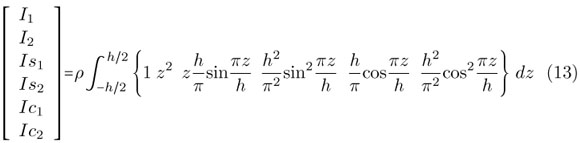3 ILLUSTRATIVE EXAMPLES

Simply supported orthotropic square and rectangular plates occupying the region given by the Eqn. (1) are considered for numerical study. The governing differential equations (7) and the associated boundary conditions (8 and 9), in terms of displacement variables, for free vibration of square and rectangular plates under consideration are as follows: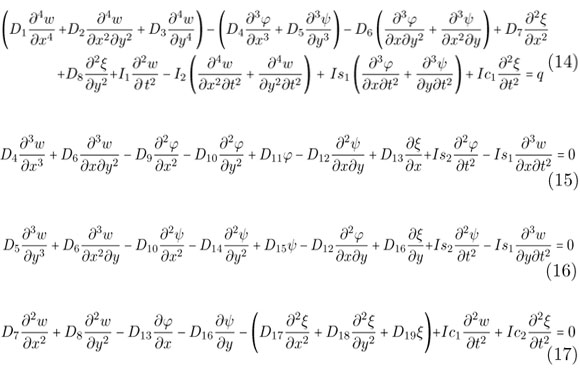The associated boundary conditions on edges x = 0 and x = a are as follows: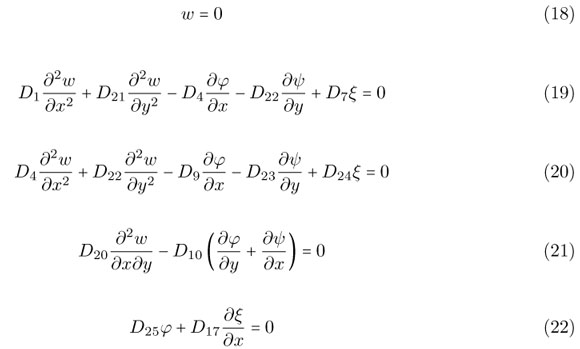The associated boundary conditions on edges y = 0 and y = b are as follows:where D1 through D27 are the stiffeness coeficients as given in Appendix.

3.1 The solution scheme

The governing equations for free flexural vibration of orthotropic plate can be obtained by setting the applied transverse load equal to zero in Eq. (14). A solution to resulting governing equations, when expressed in terms of displacement variables, which satisfies the associated boundary conditions (time dependent), is of the following form:where wmn is the amplitude of translation and φmn, ψmn and ξmn are the amplitudes of rotation. ωmn is the natural frequency of mth and nth mode of vibration. Substitution of solution form given by Eqn. (28) into the governing equations (14-17) of free vibration of orthotropic plate results in following algebraic equations:Equations (29) through (32) can be written in the following matrix form: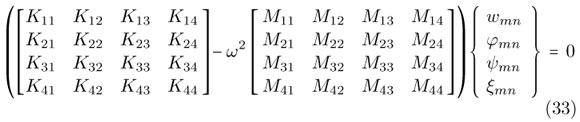(33) Eqn. (33) in more compact form can be written as follows:where [K]is the stiffness matrix, [M]is the mass matrix and {Δmn}is the vector of amplitudes of translation and rotations. The solution of Eqn. (34) is well known (see Bathe ), from this solution lowest natural frequencies for all modes of vibration can be obtained. The orthotropic plate has following material properties as given by Srinivas et al. :11 = 23.2 × 106psi,22 = 12.6 × 106psi,33 = 12.3 × 106psi,12 = 5.41 × 106psi,13 = 0.25 × 106psi,23 = 2.28 × 106psi,44 = 6.19 × 106psi,55 = 3.71 × 106psi,66 = 6.10 × 106psi,

The density (ρ) of material can be taken as any arbitrary value for calculation of frequencies.

4 NUMERICAL RESULTS

In the present paper free vibration analysis of simply supported square and rectangular orthotropic plate for aspect ratio 10 is attempted. The results obtained using present theory are compared with exact results and those of other higher order theory results available in literature wherever applicable. Following non-dimensional form is used for the purpose of presenting the results in this paper.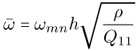The percentage error in the results obtained using a particular model with respect to the results of exact elasticity solutions is calculated as follows:4.1 Discussion of numerical results

Results obtained for frequencies of bending mode, thickness shear mode and thickness stretch mode are compared and discussed with the corresponding results of classical plate theory (CPT), first order shear deformation theory, Reddy's theory and exact theory .

A) Bending frequency (w): Table 1 shows comparison of bending frequencies for all modes of vibration for square plate (b/a = 1). It can be seen from Table 1 that the present theory yields excellent values of frequencies for all modes of vibration. The present theory, Reddy's theory  and Mindlin's theory  predicts exact result of bending frequency for fundamental mode i.e. m = 1, n = 1. Maximum percentage error predicted by present theory is 5.39 % when m = 4, n = 2 whereas maximum percentage error in Reddy's  theory is 3.50 % for the same mode of vibration. The theory of Kirchhoff (CPT) [9, 10] overestimates the fundamental bending frequency by 4.85 %.

Comparison of bending frequency for the rectangular plate (b/a =) is shown in Table 2. Because of unavailability of exact results percentage error is not quoted. From Table 2 it can be observed that present theory shows slightly lower values of bending frequency as compared to those of Reddy's  and Mindlin's  theories. In case of rectangular plate, CPT overestimates the results of bending frequency as compared to those of other higher order theories due to the neglect of transverse shear deformation and transverse normal stress effects in the classical theory.

B) Thickness shear mode frequency (φ): From the examination of Table 1 it can be observed that, for square plate (b/a = 1) the present theory gives exact values of thickness shear mode frequency for fundamental mode of vibration i.e. m = 1, n = 1 whereas theories of Reddy  and Mindlin  overestimates the same by 0.069 % and 0.63 % respectively for the same mode of vibration as compared to that of exact theory. The present theory yield excellent results for the thickness shear mode frequency for all higher modes of vibration. The theories of Reddy  and Mindlin  show higher values for the thickness shear mode frequency for all modes of vibration as compared to those of exact and present theory. The comparison of thickness shear mode frequency for rectangular plate (b/a =) as shown in Table 2. The frequencies obtained by present theory and theory of Reddy  of this plate are more or less identical with each other. However, Mindlin's  theory predicts higher values of this frequency.

Dynamic shear correction factor is the most important parameter in the dynamic analysis of plates. The exact value of this factor is given by Lamb . Present theory yields the exact value of dynamic shear correction factor (π2/12) from the circular frequency of thickness shear motion (m = 0, n = 0) for infinitely long thin rectangular plate.

C) Thickness shear mode frequency (ψ): From Table 1 it is observed that, for square plate (b/a = 1) present theory predicts exact result of this frequency for fundamental mode whereas Reddy  and Mindlin  theories overestimate the same. The theories of Reddy  and Mindlin  shows less accuracy of results for higher modes as compared to those of present and exact theories. The comparison of frequency of thickness shear mode for rectangular plate (b/a =) is shown in Table 2.

D) Thickness stretch mode frequency (ξ): In Table 1 and 2 results of frequency of thickness stretch mode of vibration are given for square and rectangular plates. The results of this frequency by other higher order theories are not available in the literature due to the neglect of transverse normal strain effect in these theories. The lowest natural frequency for this mode occurs at m = 1 and n = 4 and the highest natural frequency occurs at m = 4 and n = 1.

5 CONCLUSIONS

Following conclusions are drawn from the free vibration analysis thick orthotropic plates using variationally consistent trigonometric shear deformation theory.

1. The frequencies obtained by the present theory for bending and thickness shear modes of vibration for all modes of vibration are in excellent agreement with the exact values of frequencies for the square plate (b/a = 1).

2. The frequencies of bending and thickness shear modes of vibration according to present theory are in good agreement with those of higher order shear deformation theory for rectangular plate (b/a =) and these results are rarely available in the literature.

3. The present theory is capable to produce frequencies of thickness stretch mode of vibration.

4. The present theory yields the exact value of dynamic shear correction factor from the thickness shear motion of vibration.

In revised form 17 May 2011

APPENDIX

The stiffness coefficients D1 through D27 appeared in governing equations (14-17) and boundary conditions (18-27) are as follows.The elements of stiffness matrix [K] are as under:The elements of mass matrix [M] are as under:The vector {Δmn}in Eqn, (34) is defined as:•  K.J. Bathe. Finite Element Procedures Prentice Hall of India Pvt. Ltd., New Delhi, 1996.
•  A. Bhimaraddi and L. K. Stevens. A higher order theory for free vibration of orthotropic, homogenous, and laminated rectangular plates. ASME Journal of Applied Mechanics, 51(1):195198, 1984.
•  E. F. F. Chladni. Die Akustik Leipzig, 1802.
•  Y.M. Ghugal and R.P. Shimpi. A review of refined shear deformation theories for isotropic and anisotropic laminated plates. Journal of Reinforced Plastics and Composites, 21:775813, 2002.
•  N.F. Hanna and A.W. Leissa. A higher order shear deformation theory for the vibration of thick plates. Journal of Sound and Vibration, 170:545555, 1994.
•  R.M. Jones. Mechanics of Composite Materials Scripta Book Co., Washington, D.C., 1975.
•  T. Kant. Numerical analysis of thick plates. Computer methods in Applied Mechanics and engineering, 31(1):118, 1982.
•  W. Kim and J.N. Reddy. Novel mixed finite element models for nonlinear analysis of plates. Latin American Journal of Solids and Structures, 7:201226, 2010.
•  G.R. Kirchhoff. Uber das gleichgewicht und die bewegung einer elastischen scheibe. Journal of Reine Angew. Math. (Crelle), 40(51-88), 1850.
•  G.R. Kirchhoff. Uber die uchwingungen einer kriesformigen elastischen scheibe. Poggendorffs Annalen, 81:58264, 1850.
•  H. Lamb. On waves in an elastic plate. In Proceedings of the Royal Society of London, volume 93, pages 114128, England, 1917. Series A.
•  M. Levinson. An accurate, simple theory of the statics and dynamics of elastic plates. Mechanics: Research Communications, 7:343350, 1980.
•  M. Levy. Memoire sur la theorie des plaques elastique planes. Journal des Mathematiques Pures et Appliquees, 30:219306, 1877.
•  L. Librescu. Elastostatic and Kinetics of anisotropic and Heterogeneous shell-Type structures Noordhoff International, Leyden, The Netherlands, 1975.
•  K.M. Liew, Y. Xiang, and S. Kitipornchai. Research on thick plate vibration: a literature survey. Journal of Sound and Vibration, 180:163176, 1995.
•  K.H. Lo, R.M. Christensen, and E.M. Wu. A high-order theory of plate deformation, Part 1: homogeneous plates. ASME Journal of Applied Mechanics, 44:663668, 1977.
•  K.H. Lo, R.M. Christensen, and E.M. Wu. A high-order theory of plate deformation, Part 2: Laminated plates. ASME Journal of Applied Mechanics, 44:669676, 1977.
•  R.D. Mindlin. Influence of rotatory inertia and shear on flexural motions of isotropic, elastic plates. ASME Journal of Applied Mechanics, 18:3138, 1951.
•  A.V. Krishna Murty. Higher order theory for vibrations of thick plates. AIAA Journal, 15(12):18231824, 1977.
•  R.B. Nelson and D.R. Lorch. A refined theory for laminated orthotropic plates. ASME Journal of Applied Mechanics, 41:177183, 1974.
•  A.K. Noor and W.S. Burton. Assessment of shear deformation theories for multilayered composite plates. Applied Mechanics Reviews, 42:113, 1989.
•  L. Rayleigh. Theory of sound, volume I. Macmillan, London, 1877. reprinted 1945 by Dover, New York.
•  J.N. Reddy. A refined nonlinear theory of plates with transverse shear deformation. International Journal of Solids and Structures, 20(9/10):881896, 1984.
•  E. Reissner. The effect of transverse shear deformation on the bending of elastic plates. ASME Journal of Applied Mechanics, 12:6977, 1945.
•  W. Ritz. Uber eine neue method zur losung gewisser variations problem der mathematischen physic. Journal fur Reine und Angewandte Mathematik, 135:1161, 1909.
•  R.P. Shimpi and H.G. Patel. A two variable refined plate theory for orthotropic plate analysis. International Journal of Solids and Structures, 43:67836799, 2006.
•  S. Srinivas, C.V. Joga Rao, and A.K. Rao. An exact analysis for vibration of simply supported homogeneous and laminated thick rectangular plates. Journal of sound and vibration, 12(2):187199, 1970.
•  M. Stein and N.J.C. Bains. Post buckling behavior of longitudinally compressed orthotropic plates with transverse shearing flexibility. AIAA Journal, 28:892895, 1990.
•  V. V. Vasil'ev. The theory of thin plates. mechanics of solids. Mekhanika Tverdogo Tela, 27:2242, 1992. (English translation from original Russian by Allerton Press, New York).
• *
Author email:

# Publication Dates

• Publication in this collection
19 Dec 2011
• Date of issue
Sept 2011